# interplot: Plot the Effects of Variables in Interaction Terms

#### 2021-02-18

Interaction is a powerful tool to test conditional effects of one variable on the contribution of another variable to the dependent variable and has been extensively applied in the empirical research of social science since the 1970s (Wright Jr 1976). Unfortunately, the nonlinear nature determines that the statistical estimate of an interactive effect cannot be interpreted as straightforward as the coefficient of a regular regression parameter. Let’s use a simple example to illustrate this point: The following model use an interaction term to test the conditional effect of Z on X’s contribution (or the conditional effect of X on Z’s contribution) to the variance of Y.

$Y = \beta_0 + \beta_1X + \beta_2Z + \beta_3X\times Z + \varepsilon.$ The contribution of X on Y is typically represented by the marginal effect:

$\frac{\partial Y}{\partial X} = \beta_1 + \beta_3Z.$

The standard error of this estimate is thus:

$\hat{\sigma}_{\frac{\partial Y}{\partial X}} = \sqrt{var(\hat{\beta_1}) + Z^2var(\hat{\beta_3}) + 2Zcov(\hat{\beta_1}, \hat{\beta_3})}.$

As Brambor, Clark, and Golder (2006, 70) indicates, the above equation suggests that its perfectly possible for the contribution of X on Y to be statistically significant for certain values of Z “even if all of the model parameters are insignificant.” In other words, one cannot infer whether X has a meaningful conditional effect on Y simply from the magnitude and significance of either $$\beta_1$$ or $$\beta_3$$ (Ibid., 74). Instead, the conditional effect should be examined based on the marginal effect at every observed value of Z (Berry, Golder, and Milton 2012; Brambor, Clark, and Golder 2006; Braumoeller 2004).

Furthermore, scholars have noticed that the point estimations of some interaction models, especially those depending on link functions (e.g., logit and probit) may not be as reliable as the estimates of the confidence intervals (Berry, DeMeritt, and Esarey 2016). Therefore, it is even more important to accurately present and examine the boundaries established by the confidence intervals than the point estimates in the test of conditional effect. Berry, Golder, and Milton (2012) also pointed out that the substantive significance of the conditional effect highly relates to the distribution of the conditioning variable (viz., Z in the above example). They thus recommend researchers to present the frequency distribution of the conditioning variable together with the marginal effects (especially when the effect trend goes across the zero point).

More recently, Esarey and Sumner (2017) uncovered that the estimation of the marginal effects suggested by Brambor, Clark, and Golder (2006) might cause a “multiple comparison problem” and result over- or underconfidence of the confidential intervals. They thus recommended to adjust the CIs with a critical t-statistics following the Benjamini1995 procedure.

The interplot package provides a convenient way to operate and visualize above points with one or a series of plots produced by a single function. The function visualizes the changes in the coefficient of one variable in a two-way interaction term conditional on the value of the other included variable. The plot also includes simulated 95% confidential intervals of these coefficients. In the current version, the function works with ordinary linear regression models, generalized linear models (e.g., logit, probit, ordered logit, etc.), and multilevel (mixed-effects) regressions, all with complete or multiply imputed data.

Comparing to established alternatives such as effects::plot and sjplot::sjp.int, interplot provides a more user-friendly way to quickly produce plots that are easy to interpret. interplot plots the changes in the conditional coefficient of one variable in the interaction, rather than changes in the dependent variable itself as in the aforementioned functions. This approach avoids displaying interaction effects across multiple panels or multiple lines in favor of a single plot containing all the relevant information. Moreover, by outputting ggplot objects, interplot allows users to easily further customize their graphs.

This vignette purposes to illustrate how users can apply these functions to improve the presentation of the interactions in their models.

# Installation

To install:

• the latest released version: install.packages("interplot").
• the latest developing version: devtools::install_github("sammo3182/interplot").

# Basic Use

## Run a Model

This example is based on the mtcars dataset, which is drawn from the 1974 volume of the US magazine Motor Trend. The dependent variable is mileage in miles per (US) gallon (mpg), and the independent variables are the number of engine cylinders (cyl) and automobile weight in thousands of pounds (wt).

data(mtcars)  #load the data

Suppose we are interested in how automobile weight affects the relationship between of the number of engine cylinders on mileage and how the number of cylinders affects the relationship between the car’s weight and its mileage. Such conditional effects are modeled using a two-way multiplicative interaction term:

m_cyl <- lm(mpg ~ wt * cyl, data = mtcars)
summary(m_cyl)
##
## Call:
## lm(formula = mpg ~ wt * cyl, data = mtcars)
##
## Residuals:
##     Min      1Q  Median      3Q     Max
## -4.2288 -1.3495 -0.5042  1.4647  5.2344
##
## Coefficients:
##             Estimate Std. Error t value Pr(>|t|)
## (Intercept)  54.3068     6.1275   8.863 1.29e-09 ***
## wt           -8.6556     2.3201  -3.731 0.000861 ***
## cyl          -3.8032     1.0050  -3.784 0.000747 ***
## wt:cyl        0.8084     0.3273   2.470 0.019882 *
## ---
## Signif. codes:  0 '***' 0.001 '**' 0.01 '*' 0.05 '.' 0.1 ' ' 1
##
## Residual standard error: 2.368 on 28 degrees of freedom
## Multiple R-squared:  0.8606, Adjusted R-squared:  0.8457
## F-statistic: 57.62 on 3 and 28 DF,  p-value: 4.231e-12

The coefficient of the interactive term (wt:cyl) is positive and statistically significant at .05 level. This tells us that the coefficient of wt depends on the value of cyl and vice versa; these estimated coefficients are conditional. It does not indicate anything, however, about the magnitude or statistical significance of these conditional coefficients. A plot produced by interplot easily and clearly answers these latter questions.

## Create a Basic Graph of the Conditional Coefficients

To plot conditional coefficients, a user needs to provide only three basic pieces of information: the object of a regression result (m), the variable whose coefficient is to be plotted (var1), and the variable on which the coefficient is conditional (var2). Taking the previous example, if we intend to know how the weight of a car can affect the coefficient for number of cylinders on the mileage, var1 is cyl, and var2 is wt.

library(interplot)

interplot(m = m_cyl, var1 = "cyl", var2 = "wt")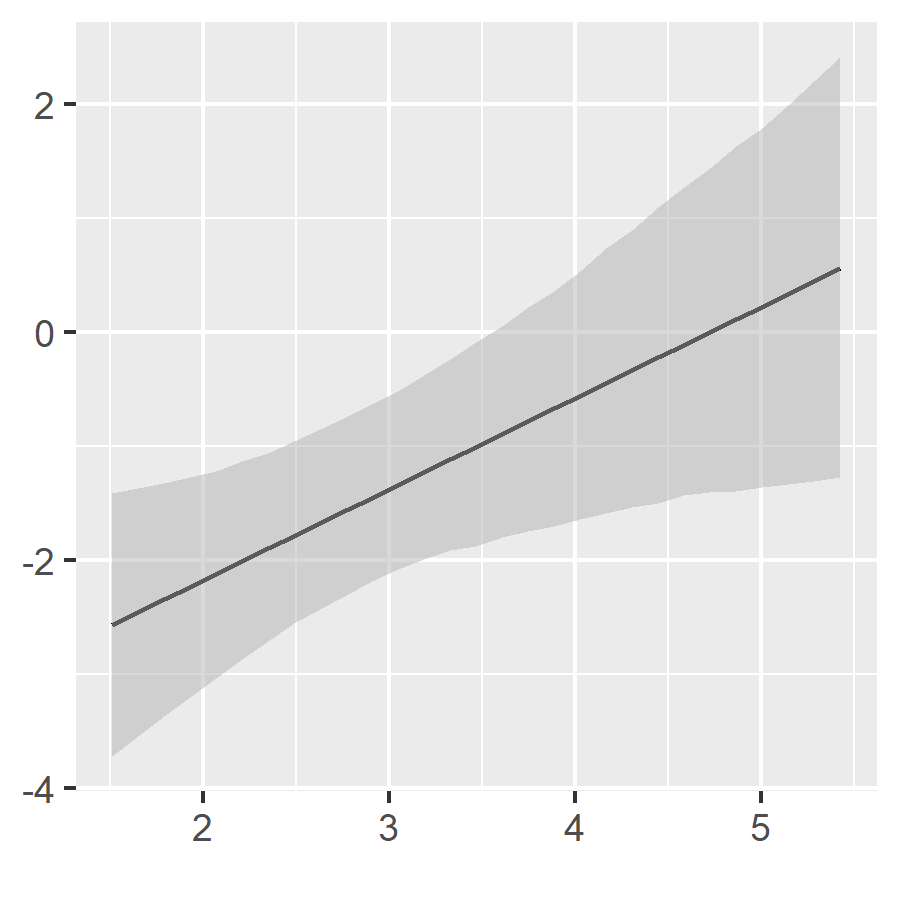The plot clearly shows that with increasing automobile weight (along the x axis), the magnitude of the coefficient of the number of cylinders on the mileage also increases (along the y axis).

Similarly, to show how the number of cylinders affects the coefficient of automobile weight on mileage, one only needs to switch var1 and var2:

interplot(m = m_cyl, var1 = "wt", var2 = "cyl")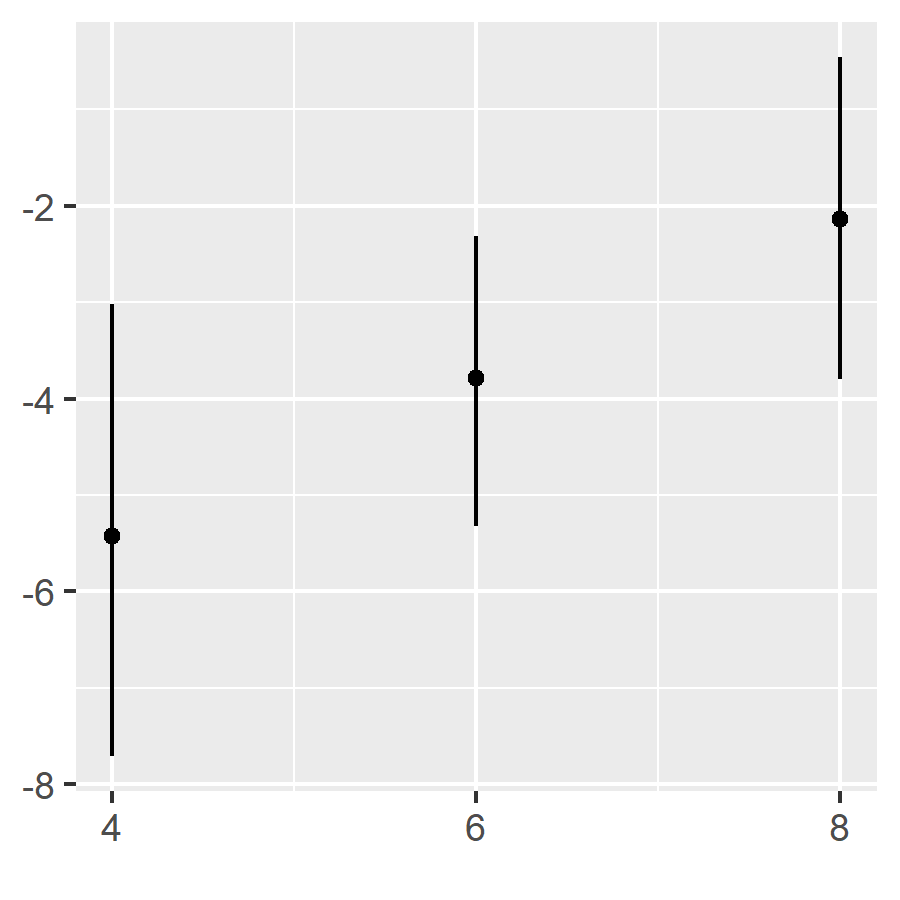Users can adjust the CI level by setting the ci option. The default value is 95% CIs. The following example resets the the CIs to 90%.

interplot(m = m_cyl, var1 = "wt", var2 = "cyl", ci = .9, point = T)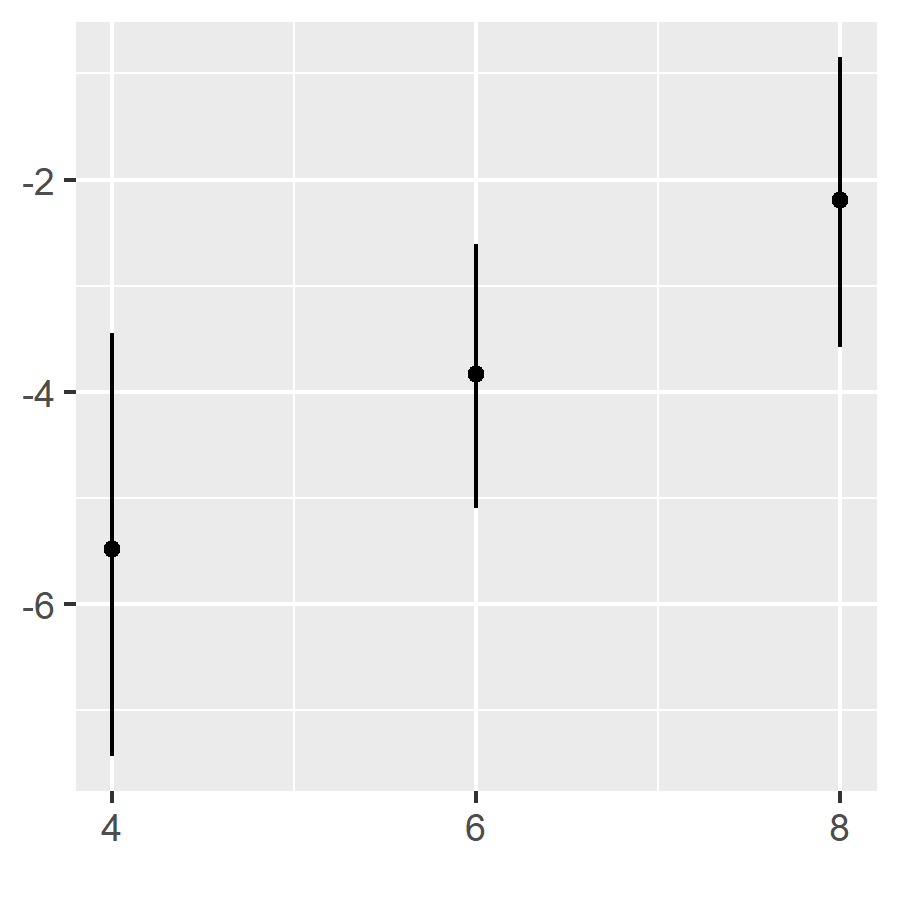The format of the plot also changes when wt is var1 and cyl is var2. This is because cyl is not a continuous variable but a categorical one with just three values: 4, 6, and 8. interplot automatically detects the number of values taken on by var2 and chooses the appropriate plot format. If there are fewer than 10 values, the function will produce a “dot-and-whisker” plot; otherwise, by default, it will generate a “line-and-ribbon” plot. Users may override this default by setting the argument point to TRUE.

interplot(m = m_cyl, var1 = "cyl", var2 = "wt", point = T) +
# changing the angle of x labels for a clearer vision
theme(axis.text.x  = element_text(angle=90))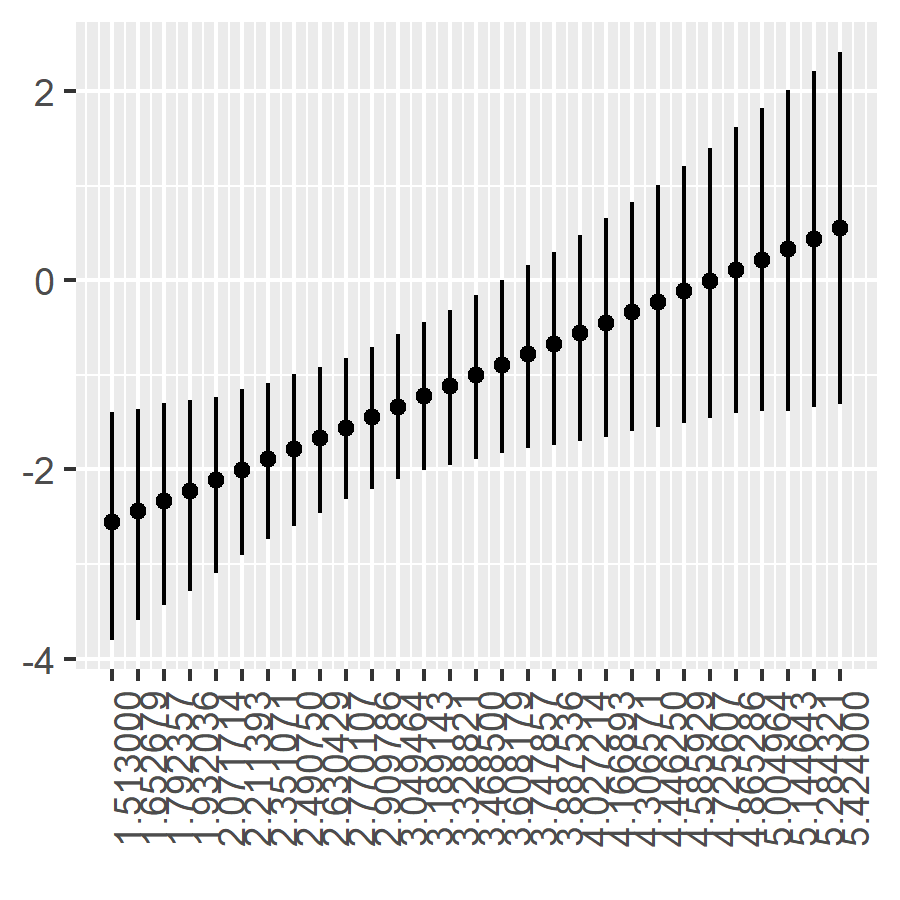## Change the Appearance of the Plot

Plots generated by interplot are, by design, very basic. They are, however, ggplot objects and so may be easily modified further.

interplot(m = m_cyl, var1 = "cyl", var2 = "wt") +
# Add labels for X and Y axes
xlab("Automobile Weight (thousands lbs)") +
ylab("Estimated Coefficient for\nNumber of Cylinders") +
# Change the background
theme_bw() +
# Add the title
ggtitle("Estimated Coefficient of Engine Cylinders \non Mileage by Automobile Weight") +
theme(plot.title = element_text(face="bold")) +
# Add a horizontal line at y = 0
geom_hline(yintercept = 0, linetype = "dashed")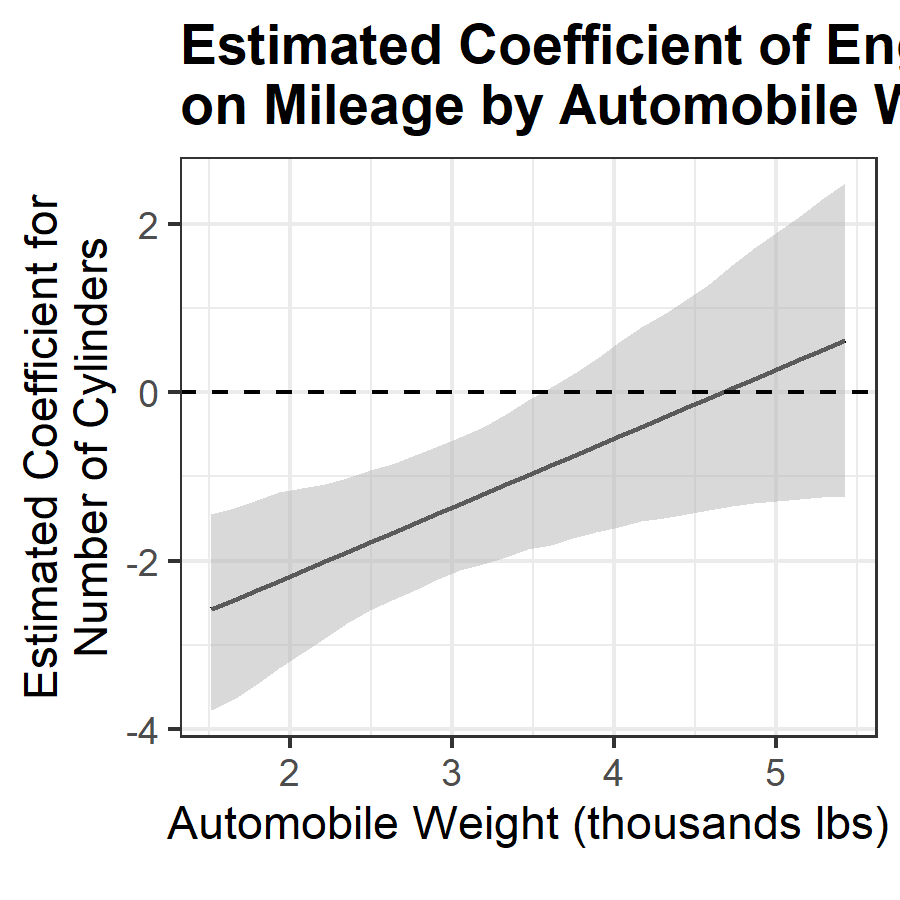For the default settings of the whisker or ribbon, the users can also use arguments, such as ercolor and esize to modify. More arguments can be found in the ?interplot file.

interplot(m = m_cyl, var1 = "wt", var2 = "cyl", ercolor = "blue", esize = 1.5) +
geom_point(size = 2, color = "red")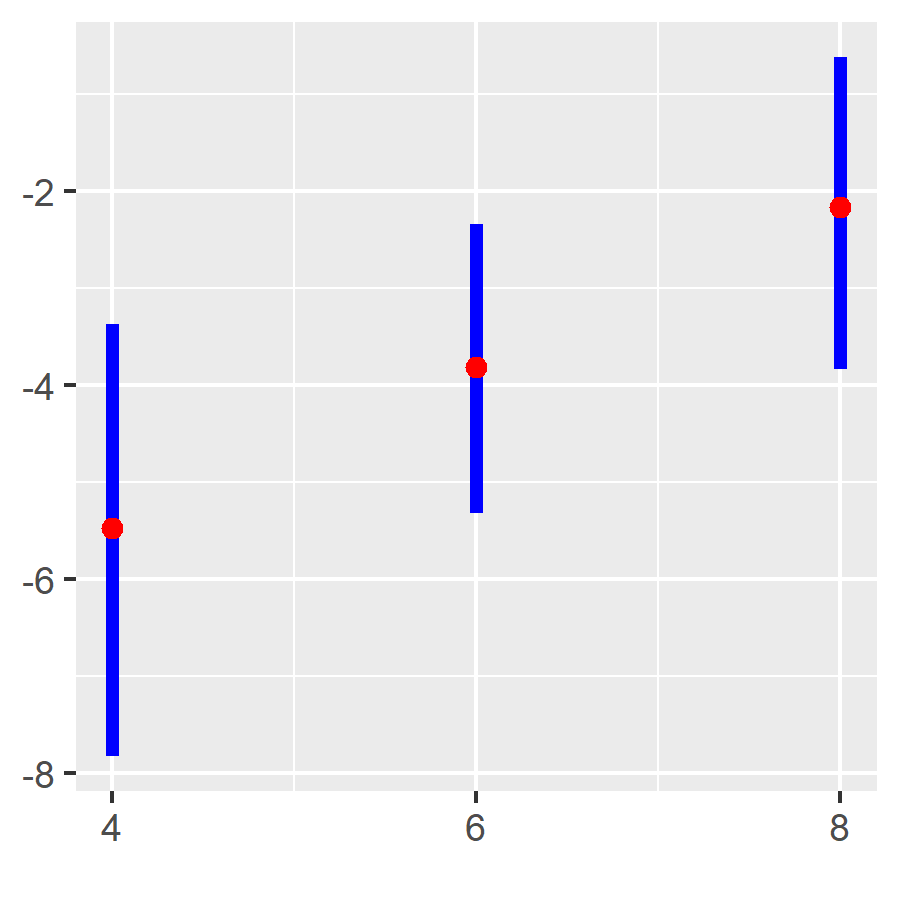## Plot the Effect of a Variable with a Quadratic Term

The simplest type of interaction is quadratic term, which can be regarded as a variable interact with itself. interplot can visualize this case when the variable names of var1 and var2 are the same.

m_wt <- lm(mpg ~ wt + I(wt^2), data = mtcars)

interplot(m = m_wt, var1 = "wt", var2 = "wt")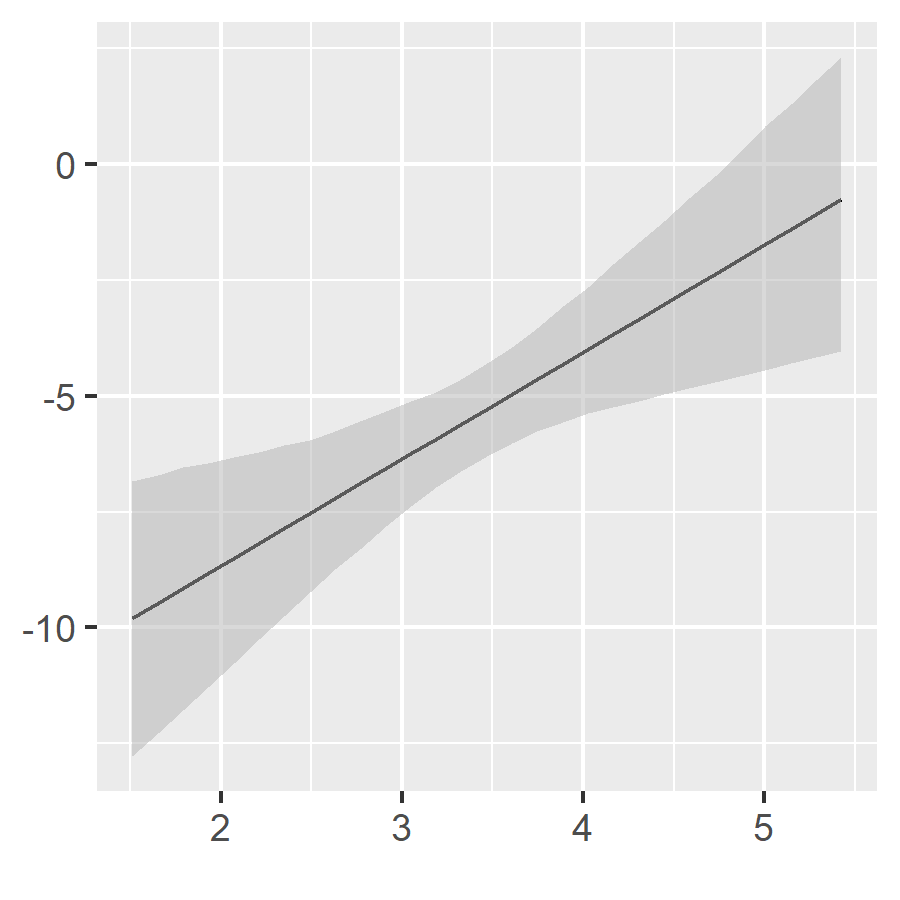## Plot Interactions with a Factor Term

When either the conditioned or the conditioning base term of an interaction is a factor, interplot creates a facet in which the conditional effect under each category of the factor is visualized in a separate panel.

mtcars$gear <- factor(mtcars$gear)
m_gear <- lm(mpg ~ gear * wt, data = mtcars)

interplot(m = m_gear, var1 = "wt", var2 = "gear")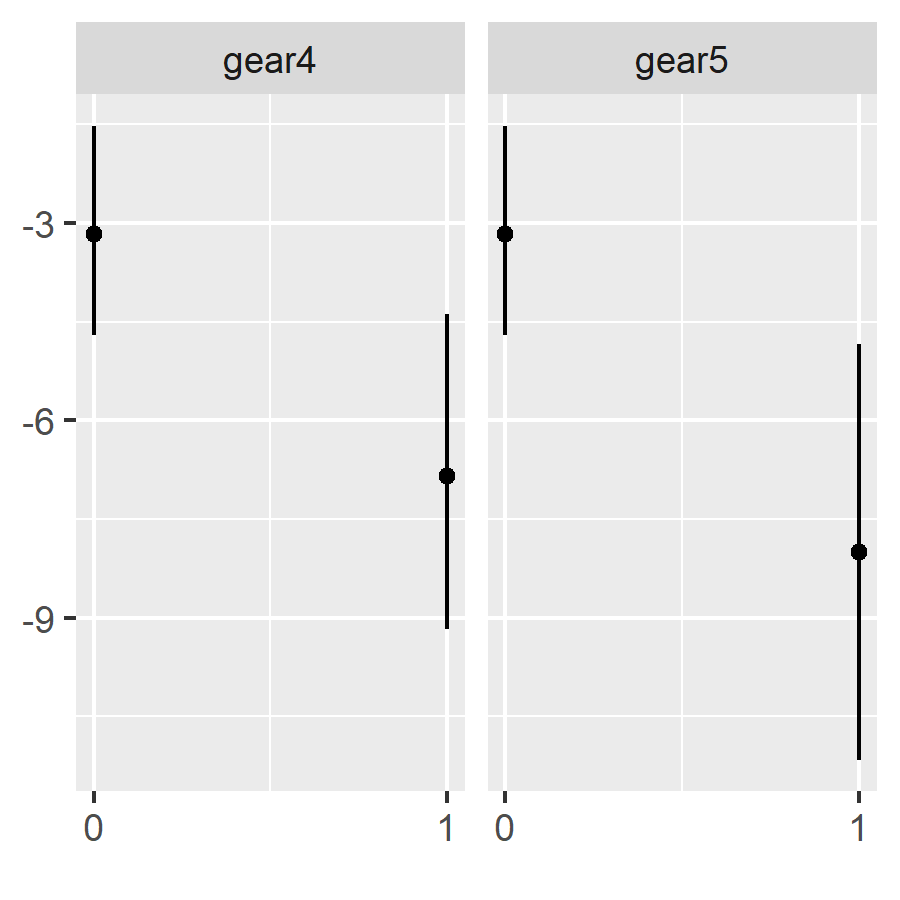To edit the facet labels, use the facet_labs argument to specify a character vector of the desired labels:

interplot(m = m_gear, var1 = "wt", var2 = "gear", facet_labs = c("4-speed", "5-speed"))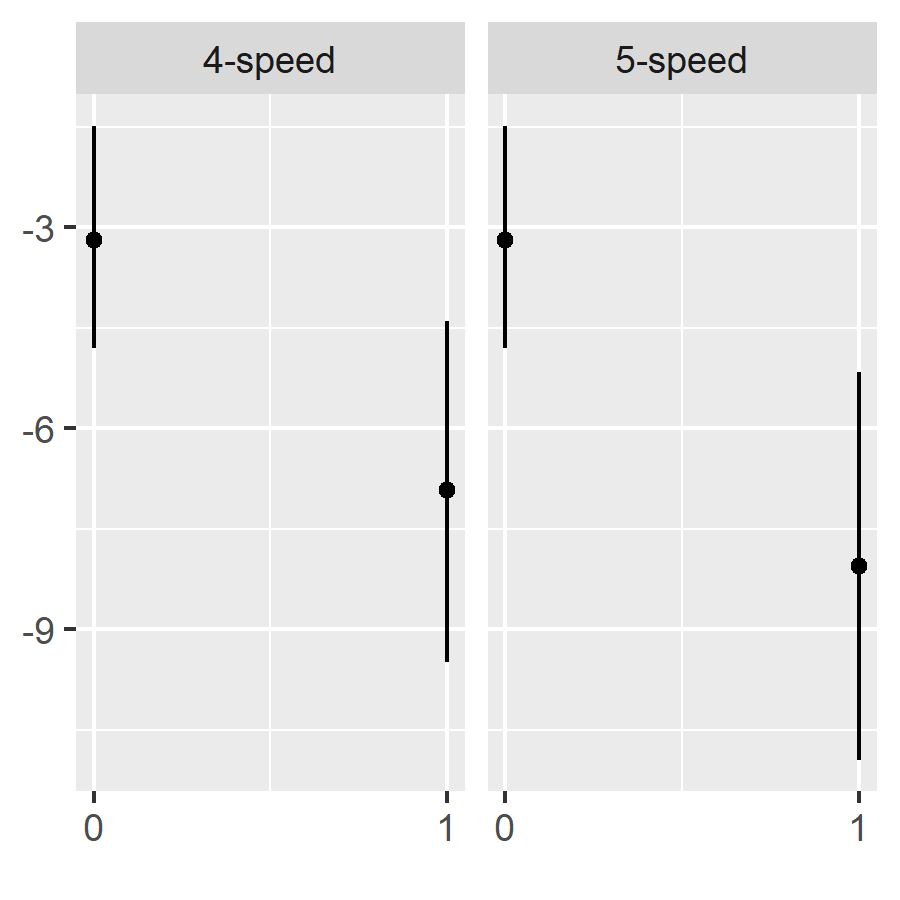## Include the Distribution of the Conditioning Variable

Berry, Golder, and Milton (2012) points out that, when a variable’s conditional effect reaches statistical significance over only part of the range of the conditioning variable, it can be helpful to the evaluation of the substantive significance of the conditional effect to know the distribution of the conditioning variable. For this purpose, interplot has the hist argument for users to choose to superimpose a histogram at the bottom of the conditional effect plot.

interplot(m = m_cyl, var1 = "cyl", var2 = "wt", hist = TRUE) +
geom_hline(yintercept = 0, linetype = "dashed")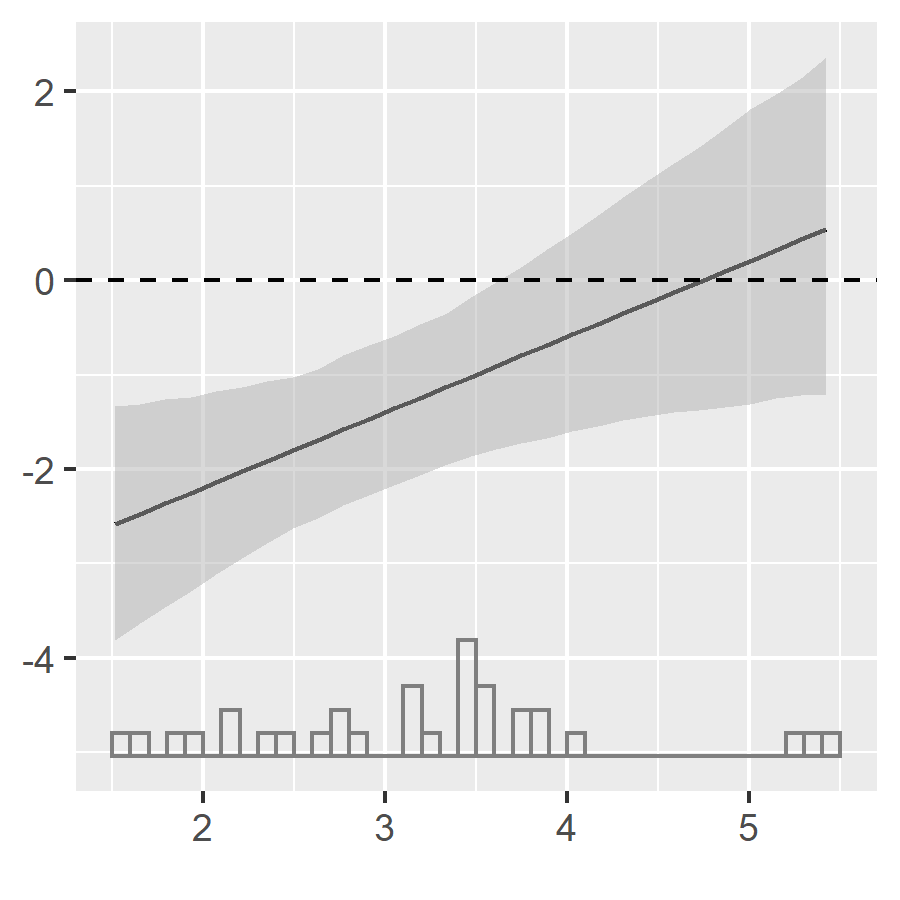Our implementation of this option was inspired by the excellent work of Hainmueller, Mummolo, and Xu (2016). A tip is that when presenting the histogram, some default setting would not be directly modified by the build-in arguments or the geom functions. Instead, one can change these settings by the aes function—as illustrated by the following example.

interplot(m = m_cyl, var1 = "cyl", var2 = "wt", hist = TRUE) +
aes(color = "pink") + theme(legend.position="none") +  # geom_line(color = "pink") +
geom_hline(yintercept = 0, linetype = "dashed")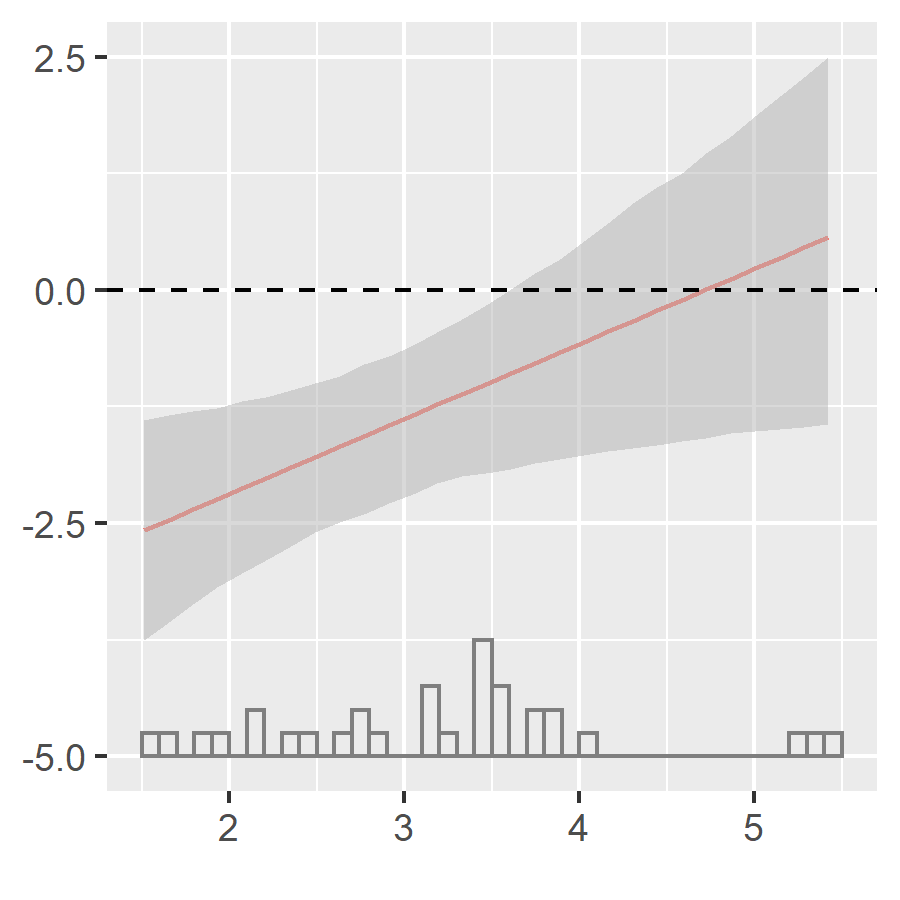# Advanced Use and Customization

## Statistical Test of the Conditional Effect

interplot visualizes the conditional effect based on simulated marginal effects. The simulation provides a probabilistic distribution of moderation effect of the conditioning variable (var2) at every preset values (including the minimum and maximum values) of the conditioned variable (var1), denoted as Emin and Emax. This output allows the function to further examine the conditional effect statistically in two ways. One is to examine if the distribution of (Emax - Emin) covers zero. The other is to directly compare Emin and Emax through statistical tools for distributional comparisons. Users can choose either method by setting the argument stats_cp to “ci” or “ks.”

• “ci” provides the confidence interval of the difference of (Emax - Emin). An interval including 0 suggests no statistical difference before and after the conditional effect is applied, and vise versa.
• “ks” presents the result of a two-sample Kolmogorov-Smirnov test of the simulated distributions of Emin and Emax. The output includes a D statistics and a p-value of the null hypothesis that the two distributions come from the same distribution at the 0.05 level.
set.seed(313)

interplot(m = m_cyl, var1 = "cyl", var2 = "wt", stats_cp = "ci")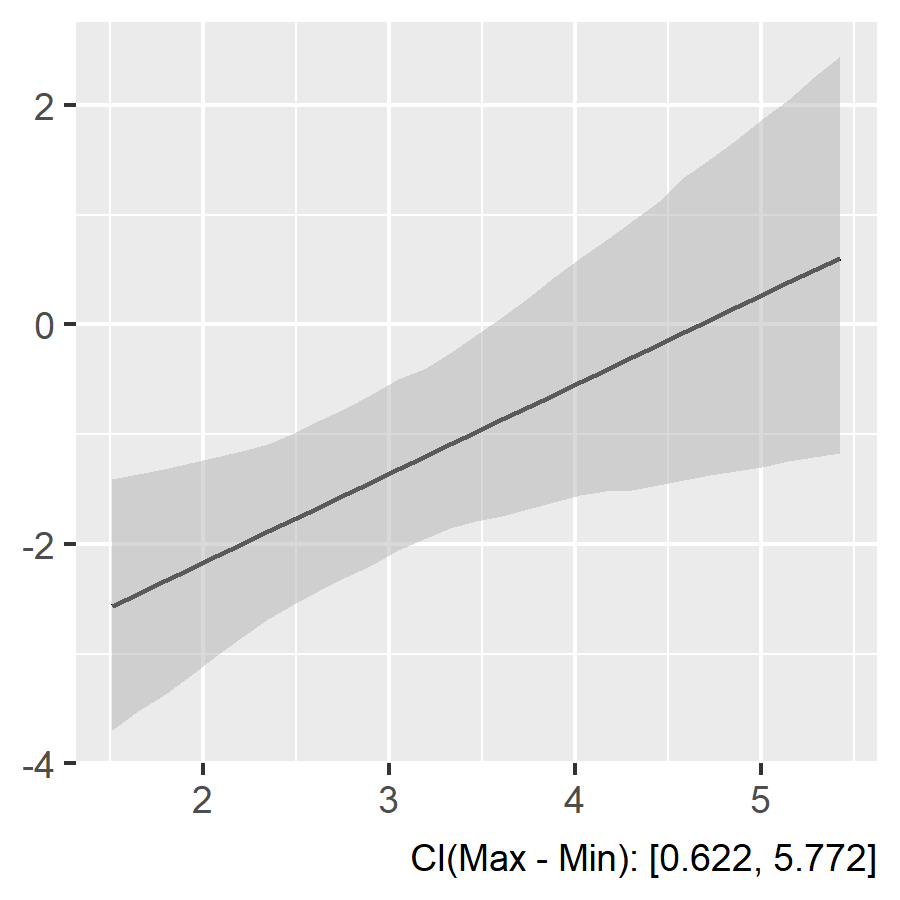interplot(m = m_cyl, var1 = "cyl", var2 = "wt", stats_cp = "ks")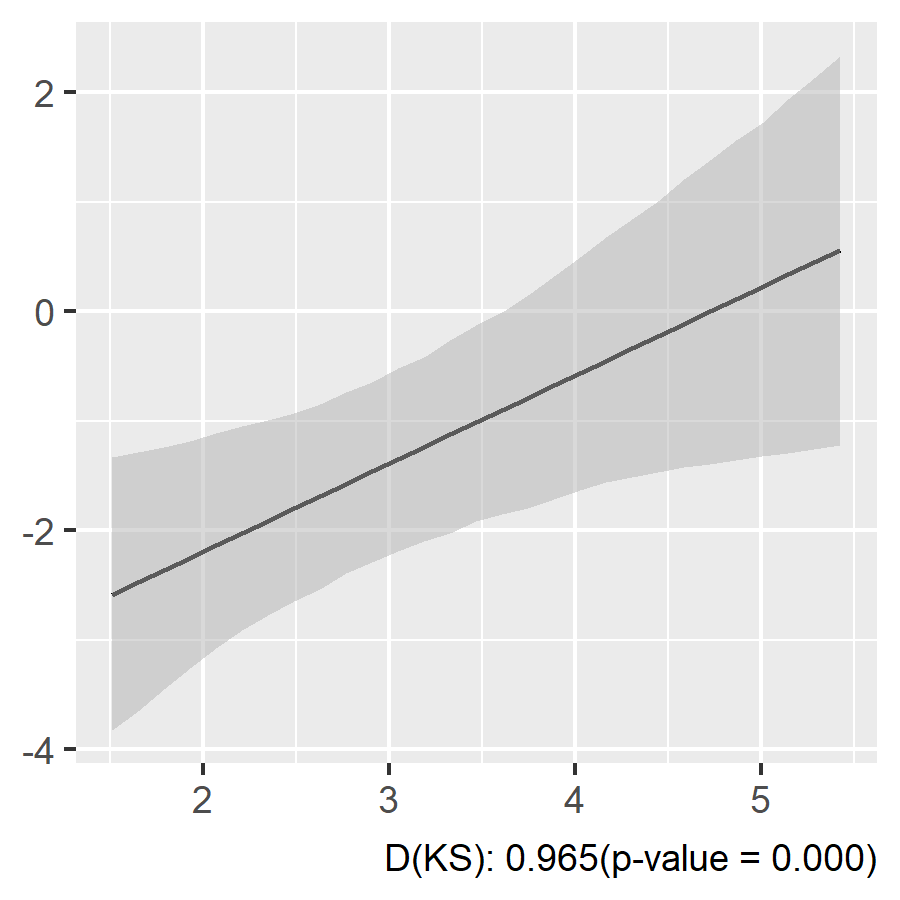since the statistical results are presented as a ggplot caption, one cannot using + lab(caption = ...) to specify it as for a regular ggplot object. Instead, users can modify the plot’s caption by specify the argument txt_caption of the dwplot function.

set.seed(313)

interplot(m = m_cyl, var1 = "cyl", var2 = "wt", stats_cp = "ci", txt_caption = "\n Source: Motor Trend Car Road Tests 1973.")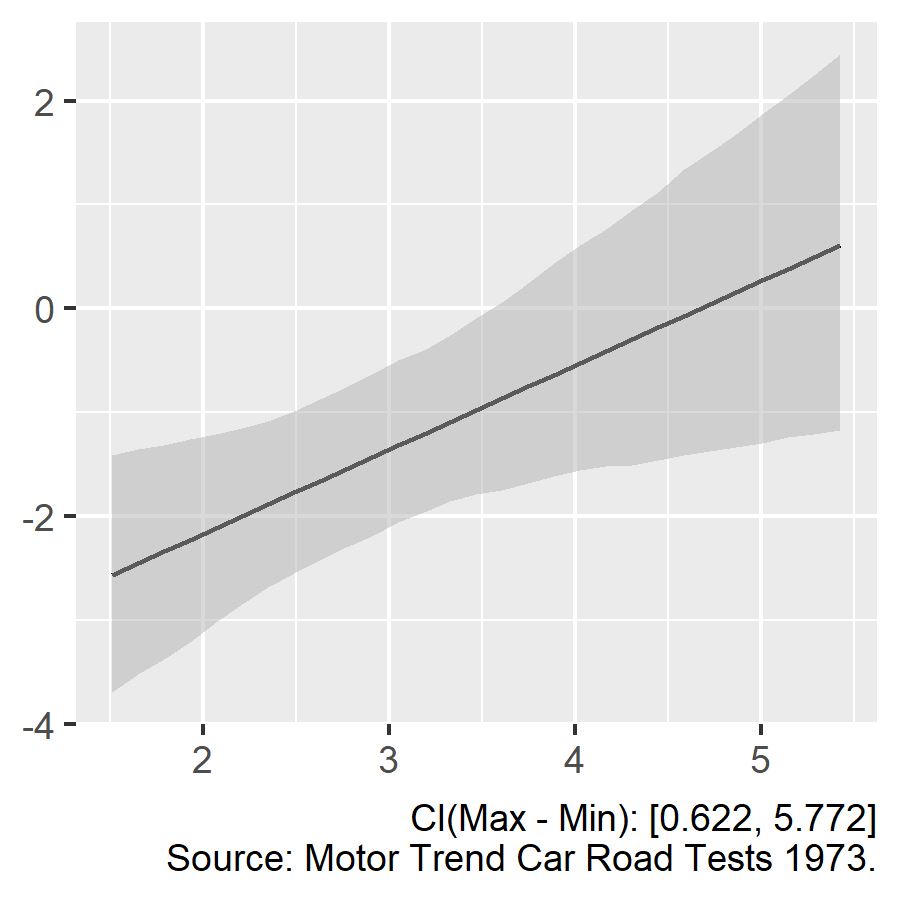## Adjust the Overconfidence in Confidence Interval Estimations

Berry, DeMeritt, and Esarey (2016) emphasized the importance to estimate the uncertainty in studying conditional effects. The most common way to do that is to follow the straightforward method of Brambor, Clark, and Golder (2006). Nevertheless, Esarey and Sumner (2017) pointed out that this method might cause a “multiple comparison problem” and result over- or underconfidence of the confidential intervals. For the overconfidence cases, they recommended to adjust the CIs with a critical t-statistics following the Benjamini1995 procedure.

interplot incorporates this recommendation with an argument adjCI. If it is set TRUE, the function will calculate the critical t-statistics to limit the false discovery rate and adjust the estimation of confidence intervals. In the following example, the left panel presents the conditional effect plot with CIs estimated based on the Brambor, Clark, and Golder (2006) method, and the right panel presents results with adjusted CIs. Although the adjustment does not change the substantial conclusion, we can see the adjusted CIs cover more area both in the middle and at the extreme values of x.

stdCI_plot <- interplot(m = m_wt, var1 = "wt", var2 = "wt", adjCI = FALSE) +
ggtitle("Marginal Effects with Standard CIs")
adjCI_plot <- interplot(m = m_wt, var1 = "wt", var2 = "wt", adjCI = TRUE) +
ggtitle("Marginal Effects with Adjusted CIs")

library(gridExtra)
grid.arrange(stdCI_plot, adjCI_plot, ncol = 2)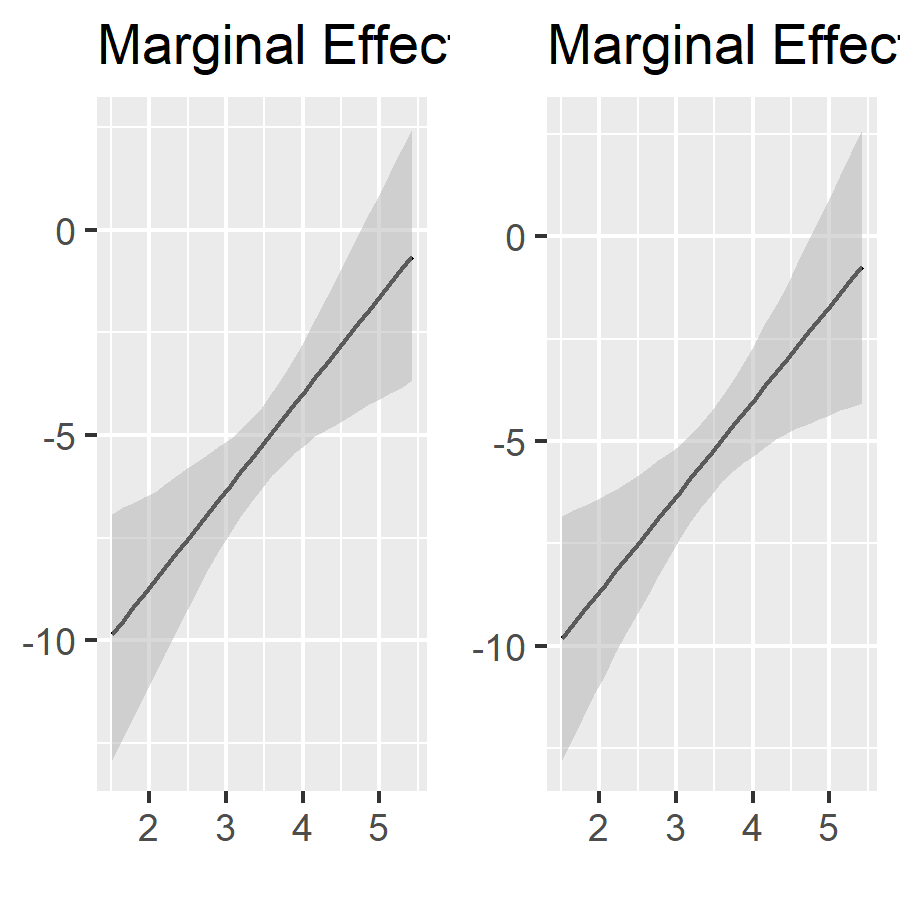## Plot Conditional Predicted Probability

Hanmer and Ozan Kalkan (2013) point out (also in the associated post in the AJPS website) that it is important to translate statistical results into meaningful quantities. The task not only pushes researchers to interpret the results in a real-life manner—which may lead to substantively different conclusions—but also provides convenience for a far broader scope of readers (e.g., policy makers, governmental officials, stakeholders, etc.) to understand the implications of the study and their importance.

To accomplish this task requires researchers to go beyond estimating the effect for the “average case,” but focuses more on the values or intervals that are illustratively important and meaningful. This could be a time-consuming job, though, especially when researchers work on models with limited dependent variables. interplot provides a convenient way to achieve the task. When researchers are dealing with general linear flat or multilevel models, they have the choice to set the argument predPro to TRUE and give the critical values they are interested in the argument var2_vals. Then, interplot will automatically estimated the conditional effects of predicted probabilities at these given values of the conditioned variable. The following example illustrates how it works.

In this example, we are interested how the economic inequality affect the impact of income on the U.S. citizens’ belief in meritocracy, a critical ideology of the “American Dream.” We estimated this conditional effect based on an interaction model with three years of Pew surveys (2006, 2007, and 2009), in which the income is the conditioned variable and economic inequality (county-level Gini coefficients).1 We first estimate the average conditional effect for the entire sample and then estimate the conditional predicted probabilities for citizens with the lowest and highest levels of income separately. As shown in the following plot, the average case in the left panel only shows a decreasing conditional effect, while the predicted probability plot in the right uncovers conditional effects in opposite directions for the high and low income individuals.

pew1.w <- read.csv("pew1_w.csv")

m <- glm(formula=meritocracy~ginicnty+income_i+ginicnty:income_i+income_cnty+black_cnty+
perc_bush04+pop_cnty+educ_i+age_i+gender_i+unemp_i+union_i+partyid_i+
ideo_i+attend_i+survid2006+survid2007+survid2009,
data=pew1.w,family=binomial(link="logit"))

plot_avg <- interplot(m, var1 = "ginicnty",var2 = "income_i", predPro = FALSE) +
ggtitle("Average Conditional Effects")

plot_3val <- interplot(m, var1 = "ginicnty",var2 = "income_i", predPro = TRUE, var2_vals = c(min(pew1.w$income_i), max(pew1.w$income_i))) +
ggtitle("Conditional Predicted Probabilities for \nCitizens with Low and High Incomes") +
scale_colour_discrete(guide = guide_legend(title = "Income"), labels = c("Low", "High")) +
scale_fill_discrete(guide = guide_legend(title = "Income"), labels = c("Low", "High")) +
theme(legend.position = c(0, .8), legend.justification = c(0, .5))

grid.arrange(plot_avg, plot_3val, ncol = 2)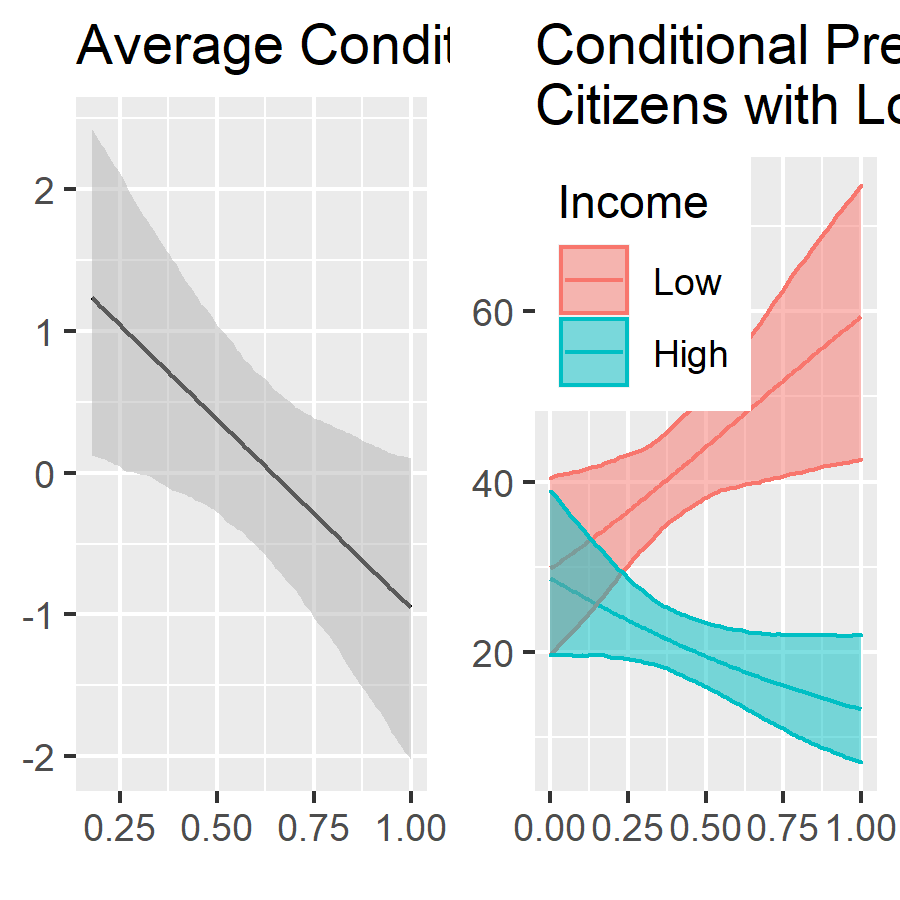## Plot Conditional Effects Without a Model

In some cases, one may analyze some complicated or self-made regression functions which are not supported by the current version of interplot. For such models, as long as the user has a dataset loading the simulated results of the interaction effects, she can still use interplot to visualize it. The dataset needs four columns the scale of the conditioning variable (fake), the simulated interactive effect at each break of the conditioning variable (coef1), and the simulated lower bound and upper bound of the confidence interval (lb, and ub). The column names should be exactly the ones shown in the above parentheses. Here is an example with some arbitrary artificial data:

# Create a fake dataset of conditional effects
fake <- rnorm(100, 0, 1)
coef1 <- fake * sample(.5:2.5, 100, replace = T)
lb <- coef1 - .5
ub <- coef1 + .5

df_fake <- data.frame(cbind(fake, coef1, lb, ub))

# Use interplot directly with the dataset
interplot(df_fake)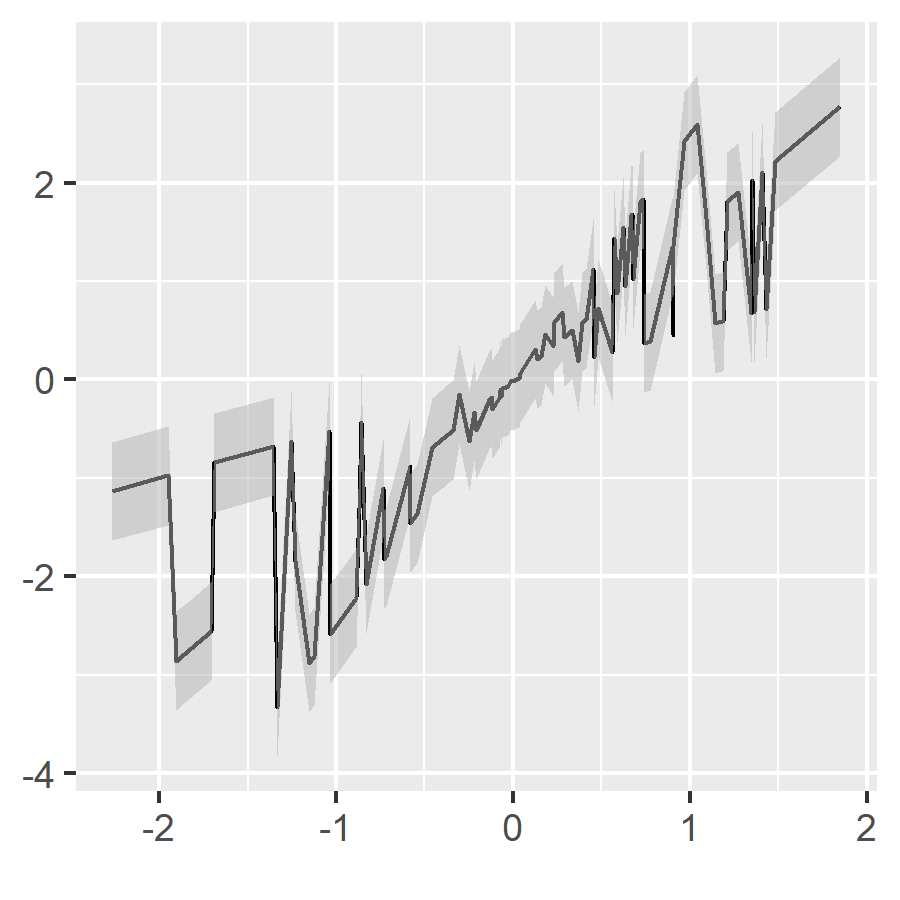If one also has the data of the var2, she can also draw a histogram under it by the argument var_dt.

var2_fake <- fake
# Set hist to TRUE is required to superimpose a histogram.
interplot(df_fake, hist = TRUE, var2_dt = var2_fake)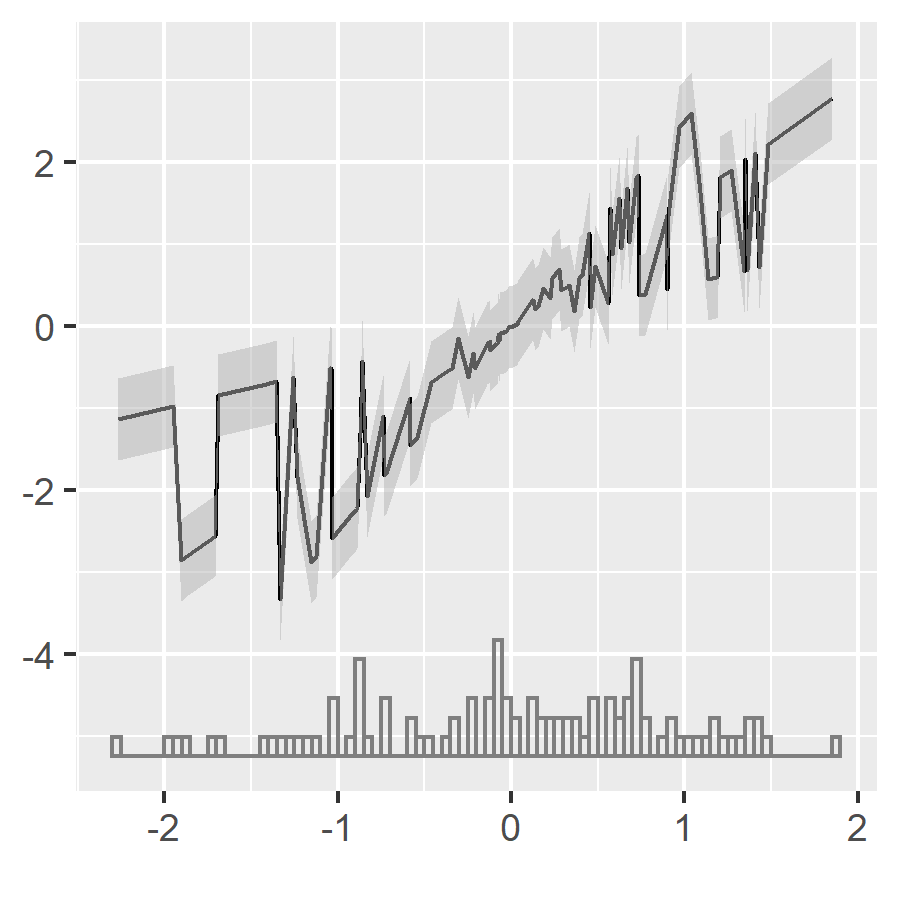## The ribbon and histogram do not fit. This is just an illustration

## Conclusion

The interplot package provides a flexible and convenient way to visualize conditional coefficients of variables in multiplicative interaction terms. This vignette offers an overview of its use and features. We encourage users to consult the help files for more details.

The development of the package is ongoing, and future research promises a compatible tool for more types of regressions and more functions. Please contact us with any questions, bug reports, and comments.

## Affiliation

Frederick Solt

Department of Political Science,

University of Iowa,

324 Schaeffer Hall,

20 E Washington St, Iowa City, IA, 52242

Email:

Website: https://fsolt.org

Yue Hu

Department of Political Science,

Tsinghua University,

Mingzhai 114,

Qinghua Yuan, Haidian District, Beijing 100084, China

Email:

Website: https://sammo3182.github.io

## Reference

Berry, William D, Jacqueline HR DeMeritt, and Justin Esarey. 2016. “Bias and Overconfidence in Parametric Models of Interactive Processes.” American Journal of Political Science 60 (2): 521–39.
Berry, William D, Matt Golder, and Daniel Milton. 2012. “Improving Tests of Theories Positing Interaction.” Journal of Politics 74 (3): 653–71.
Brambor, Thomas, William Roberts Clark, and Matt Golder. 2006. “Understanding Interaction Models: Improving Empirical Analyses.” Political Analysis 14 (1): 63–82.
Braumoeller, Bear F. 2004. “Hypothesis Testing and Multiplicative Interaction Terms.” International Organization 58 (4): 807–20.
Esarey, Justin, and Jane Lawrence Sumner. 2017. “Marginal Effects in Interaction Models: Determining and Controlling the False Positive Rate.” http://jee3.web.rice.edu/interaction-overconfidence.pdf.
Hainmueller, Jens, Jonathan Mummolo, and Yiqing Xu. 2016. “How Much Should We Trust Estimates from Multiplicative Interaction Models? Simple Tools to Improve Empirical Practice.” https://www.ssrn.com/abstract=2739221.
Hanmer, Michael J., and Kerem Ozan Kalkan. 2013. “Behind the Curve: Clarifying the Best Approach to Calculating Predicted Probabilities and Marginal Effects from Limited Dependent Variable Models.” American Journal of Political Science 57 (1): 263–77. https://doi.org/10.1111/j.1540-5907.2012.00602.x.
Solt, Frederick, Yue Hu, Kevan Hudson, Jungmin Song, and Dong Erico Yu. 2017. “Economic Inequality and Belief in Meritocracy in the United States.” Research & Politics 3 (4): 1–7. https://doi.org/10.1177/2053168016672101.
Wright Jr, Gerald C. 1976. “Linear Models for Evaluating Conditional Relationships.” American Journal of Political Science, 349–73.

1. For the purpose of illustration, we omitted the county level variance in this example. One can see more comprehensive analyses in Solt et al. (2017).↩︎